网络流24题小结

$2.$太空飞行计划问题

题目

$W$ 教授正在为国家航天中心计划一系列的太空飞行。每次太空飞行可进行一系列商业性实验而获取利润。现已确定了一个可供选择的实验集合 $E={E_1，E_2，…，E_m}$ ，和进行这些实验需要使用的全部仪器的集合 $I={I_1，I_2，…I_n}$。实验 $E_j$ 需要用到的仪器是 $I$ 的子集 $R_j \subset I$。配置仪器 $I_k$ 的费用为 $c_k$ 美元。实验 $E_j$ 的赞助商已同意为该实验结果支付 $p_j$ 美元。$W$ 教授的任务是找出一个有效算法，确定在一次太空飞行中要进行哪些实验并因此而配置哪些仪器才能使太空飞行的净收益最大。这里净收益是指进行实验所获得的全部收入与配置仪器的全部费用的差额。

题解

$S$ 向 $X$ 集合中的点连边容量为 $p_i$ 即收入， $Y$ 集合中的点向$T$连边容量为 $c_i$ 即费用，设 $Total=\sum_{i=1}^{n} p_i$ , $Cut$ 为求出的最小割(即最大流)，那么答案为 $Total-Cut$,对应的解是最小割中的点，即增广完之后仍然可以从 $S$ 点到达的点。

$A$ 集合中所有顶点的权值之和记为 $Total$，那么 $Total – Cut$ 就是(被选的$A$集合中的顶点的权值 $-$ 被选的$B$集合中的顶点的权值)，即为我们的目标函数，记为 $A$。

$3.$最小路径覆盖问题

题目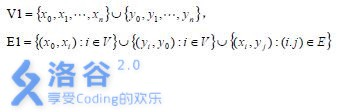题解

$S$ 先向左边集合连边，右边向 $T$ 连边，若原图中存在一条边 $(u,v)$,则建一条边 $(x[u], y[v])$， 所有边流量为 $1$，然后跑最大流出来二分图最大匹配数，点数减最大匹配数就是最少路径条数，如何输出也很简单，直接按照匹配边走就行。

$4.$魔术球问题

题目

• 每次只能在某根柱子的最上面放球。
• 在同一根柱子中，任何 $2$个相邻球的编号之和为完全平方数。

$6.$最长不下降子序列问题

题目

（1）计算其最长不下降子序列的长度s。
（2）计算从给定的序列中最多可取出多少个长度为s的不下降子序列。
（3）如果允许在取出的序列中多次使用x1和xn，则从给定序列中最多可取出多少个长度为s的不下降子序列。

$7.$试题库问题

题解

$S$ 向所有 $X$ 集合中的点连边容量为 $1$， $X$ 集合中的点分别向 $Y$ 集合中对应的类型的点连边容量为 $1$， $Y$ 集合中的点 $i$ 向 $T$ 连边容量为 $a[i]$。

$11.$航空路线问题

题目

$(1)$ 从最西端城市出发，单向从西向东途经若干城市到达最东端城市，然后再单向从东向西飞回起点(可途经若干城市)。
$(2)$除起点城市外，任何城市只能访问 $1$ 次。

$12.$软件补丁问题

题目

T 公司发现其研制的一个软件中有 $n$ 个错误，随即为该软件发放了一批共 $m$ 个补丁程序。每一个补丁程序都有其特定的适用环境，某个补丁只有在软件中包含某些错误而同时又不包含另一些错误时才可以使用。一个补丁在排除某些错误的同时，往往会加入另一些错误。

$13.$星际转移问题

题解

• 由$S$ 向 $0_n$ 连容量为 $inf$ 的边, 表示地球每天可以有无数的人移民。
• 由 $(N+1)_n$向 $T$ 连容量为 $inf$ 的边，表示月球每天可以接受无数的人。
• 对所有空间站 $i$ ，连边 $(i_{n-1}, i_n)$，容量为 $inf$，表示空间站可以让前一天的所有人今天继续留在这里。
• 对所有飞船 $x$，设其前一天在的空间站为 $stat_1$, 这一天在 $stat_2$，那么连边 $(stat_{1_{n-1}}, stat_{2_n)}$，容量为$H[x]$ ，表示飞船在这天可以将$stat_1$中的人载最多$H[x]$到$stat_2$。

$14.$孤岛营救问题

题目

$1944$ 年，特种兵麦克接到国防部的命令，要求立即赶赴太平洋上的一个孤岛，营救被敌军俘虏的大兵瑞恩。瑞恩被关押在一个迷宫里，迷宫地形复杂，但幸好麦克得到了迷宫的地形图。迷宫的外形是一个长方形，其南北方向被划分为 $N$ 行，东西方向被划分为 $M$ 列，于是整个迷宫被划分为 $N\times M$ 个单元。每一个单元的位置可用一个有序数对(单元的行号，单元的列号)来表示。南北或东西方向相邻的 $2$ 个单元之间可能互通，也可能有一扇锁着的门，或者是一堵不可逾越的墙。迷宫中有一些单元存放着钥匙，并且所有的门被分成 $P$ 类，打开同一类的门的钥匙相同，不同类门的钥匙不同。

$15.$汽车加油问题

题目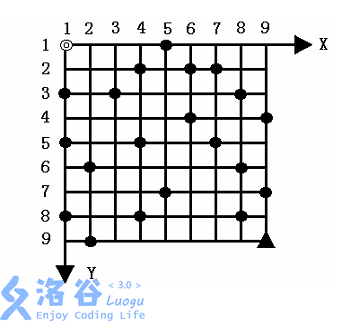1. 汽车只能沿网格边行驶，装满油后能行驶 $K$ 条网格边。出发时汽车已装满油，在起点与终点处不设油库。
2. 汽车经过一条网格边时，若其 $X$ 坐标或 $Y$ 坐标减小，则应付费用 $B$ ，否则免付费用。
3. 汽车在行驶过程中遇油库则应加满油并付加油费用 $A$ 。
4. 在需要时可在网格点处增设油库，并付增设油库费用 $C$ (不含加油费用 $A$ )。
5. $N,K,A,B,C$均为正整数， 且满足约束: $2\leq N\leq 100,2 \leq K \leq 10$。

题解

1. 如果油箱不满 $(l < K)$ ,点$i$为油库点，从 $<i,l>$ 到 $<i,K>$ 建立一条权值为$A$的有向边。
2. 如果油箱不满 $(l < K)$ ,点$i$不为油库点，从 $<i,l>$ 到 $<i,K>$ 建立一条权值为A+C的有向边。
3. 如果油箱不为空， $i$ 不为油库点，每层 $l$ 从$<i,l>$到$<j,l-1>$建立一条权值为0的有向边，其中j为i的右边或下边相邻的一个顶点；从 $<i,l>$ 到 $<j,l-1>$ 建立一条权值为B的有向边，其中j为i的左边或上边相邻的一个顶点。
4. 如果油箱不为空，$i$ 为油库点，从 $<i,K>$ 到 $<j,K-1>$ 建立一条权值为0的有向边，其中j为i的右边或下边相邻的一个顶点；从 $<i,K>$ 到 $<j,K-1>$ 建立一条权值为B的有向边，其中j为i的左边或上边相邻的一个顶点。

$16.$数字梯形问题

题目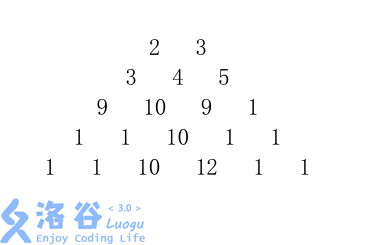1. 从梯形的顶至底的 $m$ 条路径互不相交；
2. 从梯形的顶至底的 $m$ 条路径仅在数字结点处相交；
3. 从梯形的顶至底的 $m$ 条路径允许在数字结点相交或边相交。

题解

1. 把一个点$u$拆成两个点$u_1,u_2$, $u_1$向$u_2$连边$(1,a[u])$,即流量$1$,费用（或者说是贡献）$a[u]$,若两个点之间由连边（由上向下面的两个点），就由$u_2$向$v_1$连边$(1,0)$,$S$向最上面一层$m$个点连边$(1,0)$,最下面一层点向$T$连边$(1,0)$。
2. 只需要改部分边的容量，$u_1$与$u_2$之间的边容量改为$inf$,因为点可以多次经过，最下面一层的点$v_2$与$T$的连边改为$inf$

，原因一样。

1. 所有边容量都改为$inf$,但是$S$到最上面一层点$u_1$容量为$1$，因为无论怎样最上面每个点最多且必须用一次。

$17.$运输问题

题目

W 公司有 $m$ 个仓库和 $n$ 个零售商店。第 $i$ 个仓库有 $a_i$ 单位的货物,第 $j$个零售商店需要 $b_j$ 个单位的货物。

$19.$负载平衡问题

题目

G 公司有 $n$ 个沿铁路运输线环形排列的仓库，每个仓库存储的货物数量不等。如何用最少搬运量可以使 $n$ 个仓库的库存数量相同。搬运货物时，只能在相邻的仓库之间搬运。

$20.$深海机器人问题

题目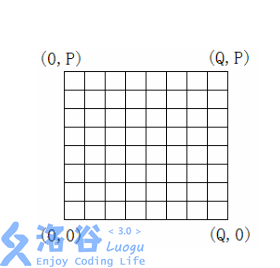题解

• 边的编号要从$2$开始，不然反边加不了，我总是忘。
• 最大费用最大流最好还是边权取相反数然后最小费用最大流再反过来靠谱一点，我直接写在松弛操作中不对。

1. 首先新建一个点$x$,连边由$u$向$x$连一条$(1,val)$，由$x$向$v$连一条$(1,0)$。
2. 再新建一个点$y$,连边由$u$向$y$连一条$(inf,0)$，由$y$向$v$连一条$(inf,0)$。

$21.$最长k可重区间集问题

题解

1、连接$S$到$S’$一条容量为$K$，费用为$0$的有向边。
2、从$S’$到每个$i.a$连接一条容量为$1$，费用为$0$的有向边。
3、从每个$i.b$到$T$连接一条容量为$1$，费用为$0$的有向边。
4、从每个顶点$i.a$到$i.b$连接一条容量为$1$，费用为区间长度的有向边。
5、对于每个区间$i$，与它右边的不相交的所有区间$j$各连一条容量为$1$，费用为$0$的有向边。

1、从$S$到顶点$1$（最左边顶点）连接一条容量为$K$，费用为$0$的有向边。
2、从顶点$2N​$（最右边顶点）到$T​$连接一条容量为$K​$，费用为$0​$的有向边。
3、从顶点$i$到顶点$i+1(i+1\leq 2N)$，连接一条容量为无穷大，费用为$0$的有向边。
4、对于每个区间$[a,b]$，从$a$对应的顶点$i$到$b$对应的顶点$j$连接一条容量为$1$，费用为区间长度的有向边。

$22.$最长k可重线段集问题

题目

$$|z|=\lfloor\sqrt{(x_1-x_0)^2+(y_1-y_0)^2}\rfloor$$

$23.$火星探险问题

题目

X 1,Y 1 X 2 , Y 1 X 3 , Y 1 … X P-1, Y 1 X P , Y 1

X 1,Y 2 X 2 , Y 2 X 3 , Y 2 … X P-1, Y 2 X P , Y 2

X 1, Y 3 X 2 , Y 3 X 3 ,Y 3 … X P-1, Y 3 X P , Y 3

… …

X 1 ,Y Q-1 X 2 , Y Q-1 X 3 , Y Q-1 … X P-1, Y Q-1 X P , Y Q-1

X 1,Y Q X 2 , Y Q X 3 , Y Q … X P-1, Y Q X P ,Y Q

$24.$骑士共存问题

题目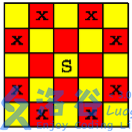题解

1. $S$向$X$集合中的没障碍的点连边容量为$1$。
2. $Y$集合中的没障碍的点向$T$连边容量为$1$。
3. 对于可以互相攻击到的两个点从一个点（假设他在$X$集合中）向另一个点（肯定在$Y$集合中）连边容量为$inf$。sys_con

OIer，常规 / 竞赛都渣得不行

2 thoughts to “网络流 24 题题解”

1.Kewth说道：

太 6 了

1.sys_con说道：

并做网络流时别人建议做的而已